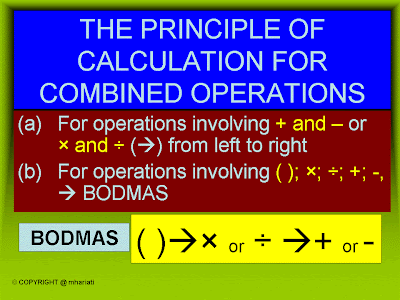## 29 November 2010

### COMBINED OPERATIONS OF INTEGERSAlways follow the rule

Example 1 :
Question (a) Involve addition and subtraction operations, so the solution is from left to right
Step 1 : Find the answer for -5 - 8 that is -13
Step 2 : Then -13 + 4 so the answer is -9

Question (b) Involve division and multiplication, so the solution is from left to right
Step 1 : Find the answer for division the answer is -6
Step 2 : Then -6 multiply -5, answer is 30

Example 2
Question (a) Involving subtraction and multiplication, the solution is BODMAS
Step 1 : Find the answer for multiplication , 3 x (-7) = -21, but make sure you write at the back just like the position in question
Step 2 : Simplify the -(-) = +
Step 3 : Lastly, calculate the addition of -15 and 21, the answer is 6

Question (b) Involving addition, division and bracket, so use BODMAS
Step 1 : First, find the answer inside bracket [2 x (-8)]= -16
Step 2 : Followed by division, (-16)/(-16) = 1
Step 3 : Lastly addition , 12 + 1 = 13

Example 3
Question (a) Involving multiplication and division but in fraction
Step 1 : Simplify until get the smallest number
Step 2 : Multiply  6 x 1 = 6
Step 3 : Lastly, 6 divide with (-1), 6/(-1) = -6

Question(b) Involving division, addition and multiplication, use BODMAS
Step 1 : Find the answer for division and multiplication, but make sure you write the position same as in the question
Step 2 : Simplify the symbol +(-) = -
Step 3: Lastly - 4 - 14 = - 18

1.Can you write it simply as you can?i don't really understand.

2.3.nice examples, but less explanation.... could you write more examples????... maybe it helps....

4.Can you more specific with example you want me give another example?

5.We absolutely love your blog and find the majority of your post's to be exactly what I'm looking for. Do you offer guest writers to write content to suit your needs? I wouldn't mind composing a post or elaborating on a number of the subjects you write concerning here. Again, awesome weblog!
My site > www.dreampharmacy-online.com

6.tq so much